Main Content

# Cumulative Product

Cumulative product of channel, column, or row elements

## Library

Math Functions / Math Operations

`dspmathops`

•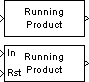## Description

The Cumulative Product block computes the cumulative product along the specified dimension of the input or across time (running product).

The input can be a vector or matrix.

### Input and Output Characteristics

Valid Input

The Cumulative Product block accepts vector or matrix inputs containing real or complex values.

Valid Reset Signal

The optional reset port, `Rst`, accepts scalar values, which can be any built-in Simulink® data type including `boolean`. The rate of the input to the Rst port must be the same or slower than that of the input data signal. The sample time of the input to the Rst port must be a positive integer multiple of the input sample time.

### Computing the Running Product Along Channels of the Input

When you set the Multiply input along parameter to `Channels (running product)`, the block computes the cumulative product of the elements in each input channel. The running product of the current input takes into account the running product of all previous inputs. In this mode, you must also specify a value for the Input processing parameter. When you set the Input processing parameter to `Columns as channels (frame based)`, the block computes the running product along each column of the current input. When you set the Input processing parameter to ```Elements as channels (sample based)```, the block computes a running product for each element of the input across time. See the following sections for more information:

Computing the Running Product for Each Column of the Input

When you set the Input processing parameter to `Columns as channels (frame based)`, the block treats each input column as an independent channel. As the following figure and equation illustrate, the output has the following characteristics:

• The first row of the first output is the same as the first row of the first input.

• The first row of each subsequent output is the element-wise product of the first row of the current input (time t), and the last row of the previous output (time t - Tf, where Tf is the frame period).

• The output has the same size, dimension, data type, and complexity as the input.

Given an M-by-N matrix input, u, the output, y, is an M-by-N matrix whose first row has elements

`${y}_{1,j}\left(t\right)={u}_{1,j}\left(t\right)\cdot {y}_{M,j}\left(t-{T}_{f}\right)$`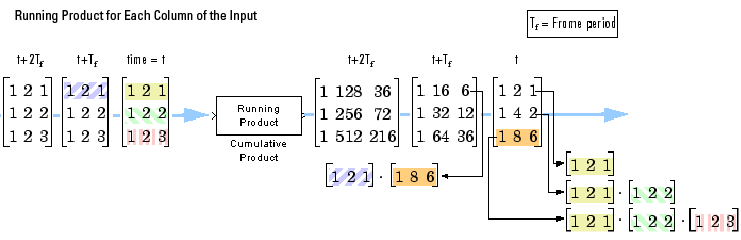Computing the Running Product for Each Element of the Input

When you set the Input processing parameter to `Elements as channels (sample based)`, the block treats each element of the input matrix as an independent channel. As the following figure and equation illustrate, the output has the following characteristics:

• The first output is the same as the first input.

• Each subsequent output is the element-wise product of the current input (time t) and the previous output (time t - Ts, where Ts is the sample period).

• The output has the same size, dimension, data type, and complexity as the input.

Given an M-by-N matrix input, u, the output, y, is an M-by-N matrix with the elements

`${y}_{i,j}\left(t\right)={u}_{i,j}\left(t\right)\cdot {y}_{i,j}\left(t-{T}_{s}\right)\text{\hspace{0.17em}}\text{\hspace{0.17em}}\begin{array}{c}1\le i\le M\\ 1\le j\le N\end{array}$`

For convenience, the block treats length-M unoriented vector inputs as M-by-1 column vectors when multiplying along channels. In such cases, the output is a length-M unoriented vector.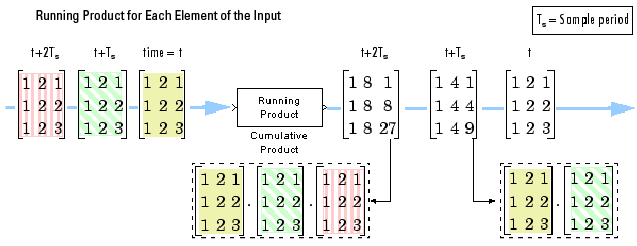Resetting the Running Product

When you are computing the running product, you can configure the block to reset the running product whenever it detects a reset event at the optional `Rst` port. The rate of the input to the Rst port must be the same or slower than that of the input data signal. The sample time of the input to the Rst port must be a positive integer multiple of the input sample time. The input to the `Rst` port can be of the Boolean data type.

If a reset event occurs while the block is performing sample-based processing, the block initializes the current output to the values of the current input. If a reset event occurs while the block is performing frame-based processing, the block initializes the first row of the current output to the values in the first row of the current input.

The Reset port parameter specifies the reset event, which can be one of the following:

• `None` disables the `Rst` port.

• `Rising edge` — Triggers a reset operation when the `Rst` input does one of the following:

• Rises from a negative value to a positive value or zero

• Rises from zero to a positive value, where the rise is not a continuation of a rise from a negative value to zero (see the following figure)• `Falling edge` — Triggers a reset operation when the `Rst` input does one of the following:

• Falls from a positive value to a negative value or zero

• Falls from zero to a negative value, where the fall is not a continuation of a fall from a positive value to zero (see the following figure)• `Either edge` — Triggers a reset operation when the `Rst` input is a ```Rising edge``` or `Falling edge` (as described above)

• `Non-zero sample` — Triggers a reset operation at each sample time that the `Rst` input is not zero

Note

When you run simulations in Simulink `MultiTasking` mode, reset signals have a one-sample latency. When the block detects a reset event, a one-sample delay occurs at the reset port rate before the block applies the reset. For more information on latency and the Simulink tasking modes, see Excess Algorithmic Delay (Tasking Latency) and Time-Based Scheduling and Code Generation (Simulink Coder).

### Multiplying Along Columns

When you set the Multiply input along parameter to `Columns`, the block computes the cumulative product of each column of the input. In this mode, the current cumulative product is independent of the cumulative products of previous inputs.

```y = cumprod(u) % Equivalent MATLAB code ```

The output has the same size, dimension, data type, and complexity as the input. The mth output row is the element-wise product of the first m input rows.

Given an M-by-N input, u, the output, y, is an M-by-N matrix whose jth column has elements

When multiplying along columns, the block treats length-M unoriented vector inputs as M-by-1 column vectors.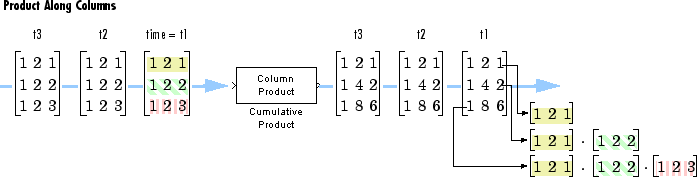### Multiplying Along Rows

When you set the Multiply input along parameter to `Rows`, the block computes the cumulative product of the row elements. In this mode, the current cumulative product is independent of the cumulative products of previous inputs.

```y = cumprod(u,2) % Equivalent MATLAB code ```

The output has the same size, dimension, and data type as the input. The nth output column is the element-wise product of the first n input columns.

Given an M-by-N matrix input, u, the output, y, is an M-by-N matrix whose ith row has elements

When you multiply along rows, the block treats length-N unoriented vector inputs as 1-by-N row vectors.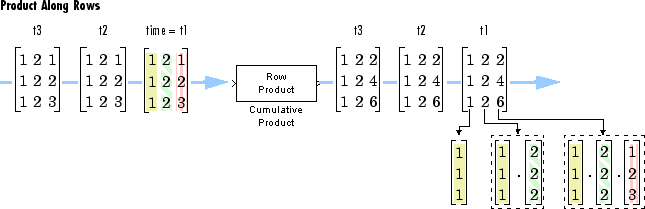### Fixed-Point Data Types

The following diagram shows the data types used within the Cumulative Product block for fixed-point signals.The output of the multiplier is in the product output data type when at least one of the inputs to the multiplier is real. When both of the inputs to the multiplier are complex, the result of the multiplication is in the accumulator data type. For details on the complex multiplication performed, see Multiplication Data Types. You can set the accumulator, product output, intermediate product, and output data types in the block dialog as discussed in Parameters.

## Parameters

Main Tab

Multiply input along

Specify the dimension along which to compute the cumulative product. You can choose to multiply along ```Channels (running product)```, `Columns`, or `Rows`. For more information, see the following sections:

Input processing

Specify how the block should process the input when computing the running product along the channels of the input. You can set this parameter to one of the following options:

• `Columns as channels (frame based)` — When you select this option, the block treats each column of the input as a separate channel.

• `Elements as channels (sample based)` — When you select this option, the block treats each element of the input as a separate channel.

This parameter is available only when you set the Multiply input along parameter to ```Channels (running product)```.

Reset port

Determines the reset event that causes the block to reset the product along channels. The rate of the input to the Rst port must be the same or slower than that of the input data signal. The sample time of the input to the Rst port must be a positive integer multiple of the input sample time. This parameter appears only when you set the Multiply input along parameter to ```Channels (running product)```. For more information, see Resetting the Running Product.

Data Types Tab

Note

Floating-point inheritance takes precedence over the data type settings defined on this pane. When inputs are floating point, the block ignores these settings, and all internal data types are floating point.

Rounding mode

Specify the rounding mode for fixed-point operations as one of the following:

• `Floor`

• `Ceiling`

• `Convergent`

• `Nearest`

• `Round`

• `Simplest`

• `Zero`

For more details, see rounding mode.

Saturate on integer overflow

When you select this parameter, the block saturates the result of its fixed-point operation. When you clear this parameter, the block wraps the result of its fixed-point operation. For details on `saturate` and `wrap`, see overflow mode for fixed-point operations.

Intermediate product

Specify the intermediate product data type. As shown in Fixed-Point Data Types, the output of the multiplier is cast to the intermediate product data type before the next element of the input is multiplied into it. You can set it to:

• A rule that inherits a data type, for example, `Inherit: Same as input`

• An expression that evaluates to a valid data type, for example, `fixdt([],16,0)`

Click the buttonto display the Data Type Assistant, which helps you set the Product output parameter.

See Specify Data Types Using Data Type Assistant (Simulink) for more information.

Product output

Specify the product output data type. See Fixed-Point Data Types and Multiplication Data Types for illustrations depicting the use of the product output data type in this block. You can set it to:

• A rule that inherits a data type, for example, `Inherit: Same as input`

• An expression that evaluates to a valid data type, for example, `fixdt([],16,0)`

Click the buttonto display the Data Type Assistant, which helps you set the Product output parameter.

See Specify Data Types Using Data Type Assistant (Simulink) for more information.

Accumulator

Specify the accumulator data type. See Fixed-Point Data Types for illustrations depicting the use of the accumulator data type in this block. You can set this parameter to:

• A rule that inherits a data type, for example, `Inherit: Same as input`

• A rule that inherits a data type, for example, ```Inherit: Same as product output```

• An expression that evaluates to a valid data type, for example, `fixdt([],16,0)`

Click the buttonto display the Data Type Assistant, which helps you set the Accumulator parameter.

See Specify Data Types Using Data Type Assistant (Simulink) for more information.

Output

Specify the output data type. See Fixed-Point Data Types for illustrations depicting the use of the output data type in this block. You can set it to:

• A rule that inherits a data type, for example, `Inherit: Same as input`

• A rule that inherits a data type, for example, ```Inherit: Same as product output```

• An expression that evaluates to a valid data type, for example, `fixdt([],16,0)`

If both inputs are unsigned, all data types including the output data type is unsigned. If one of the inputs is signed, internal and output data types are signed.

Click the buttonto display the Data Type Assistant, which helps you set the Output parameter.

See Control Signal Data Types (Simulink) for more information.

Minimum

Specify the minimum value that the block should output. The default value is `[]` (unspecified). Simulink uses this value to perform:

• Simulation range checking (see Specify Signal Ranges (Simulink))

• Automatic scaling of fixed-point data types

Maximum

Specify the maximum value that the block should output. The default value is `[]` (unspecified). Simulink uses this value to perform:

• Simulation range checking (see Specify Signal Ranges (Simulink))

• Automatic scaling of fixed-point data types

Lock data type settings against changes by the fixed-point tools

Select this parameter to prevent the fixed-point tools from overriding the data types you specify on the block mask.

## Supported Data Types

Input and Output PortsSupported Data Types

Data input port, `In`

• Double-precision floating point

• Single-precision floating point

• Fixed point

• 8-, 16-, and 32-bit signed integers

• 8-, 16-, and 32-bit unsigned integers

Reset input port, `Rst`

All built-in Simulink data types:

• Double-precision floating point

• Single-precision floating point

• Boolean

• 8-, 16-, and 32-bit signed integers

• 8-, 16-, and 32-bit unsigned integers

Output port

• Double-precision floating point

• Single-precision floating point

• Fixed point

• 8-, 16-, and 32-bit signed integers

• 8-, 16-, and 32-bit unsigned integers

## See Also

### Blocks

Introduced before R2006a

Watch now